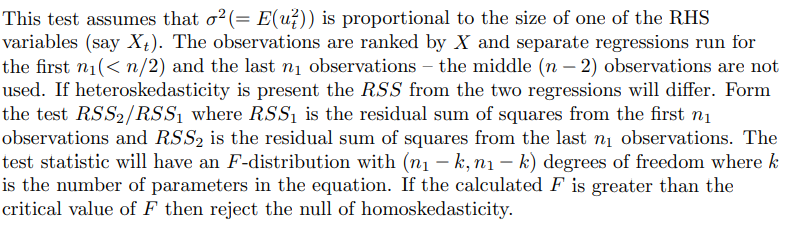# Heteroscedasticity test procedure: 5-steps method to understand Goldfield-Quandt and the White Test for Heteroscedasticity

Goldfield-QuandtStep 1:

Get the data.

Step 2:

Second step involves arranging the X1 values from lowest to highest order. Then, divide the series into two groups, n1 and n2 and remember n1, and n2 must less than N/2. n1,n2 being the size of first and second group respectively and N is the size of the original group. Here, N= 30, n1 and n2 = 13.

Step 3:

Run two regression using the values from the two groups. Check for their Residual Sum of Squares (RSS) and find out the ratios between them.

The ratio = 1328.9651/ 353.411 = 3.760

Step 4:

Compare the calculated F-Statistics(F-Cal) with critical F-value(F-Crit) with the degrees of freedom and significance level.

Step 5: Decision Rules

If F-Cal > F-Crit,

If F-Cal < F-Crit

This calculated value is larger than the critical F – value at 11 degrees of freedom with a 5 % level of significance. Reject the null hypothesis.

White Test

Step 1:

Run regression using the above model. Get the residuals and squared residuals.

Step 2:

Run an auxiliary regression using the following model:

Step 3:

Find the R-square from this auxiliary regression. Then, use it to compare with the following formula.

Step 4:

Decision Rules:

If Chi-Cal < Chi-Crit

If Chi-Cal > Chi-Crit

Step 5:

If the Chi-Cal value is greater than the Chi-Crit value then reject the null hypothesis.

Similarities

Both used for testing heteroscedasticity.

Differences

For G-Q test, its necessary to rearrange the X values and know the X having the most impact on the dependent variable. While its not necessary for white test. Assumption of normality is necessary for both.